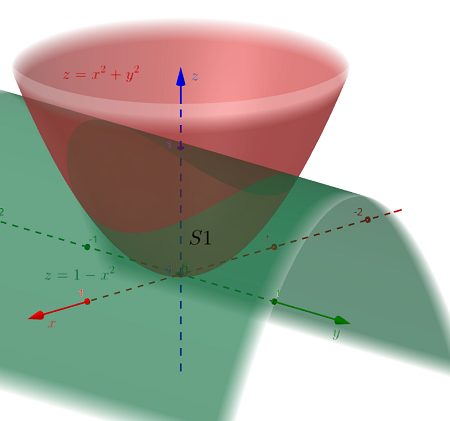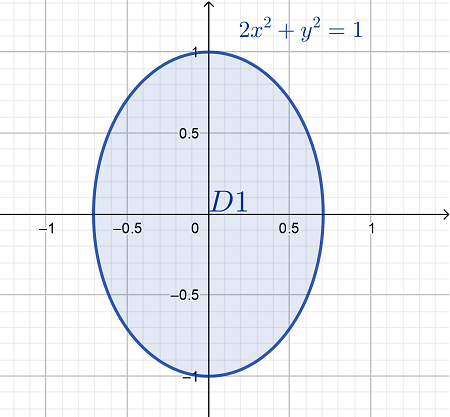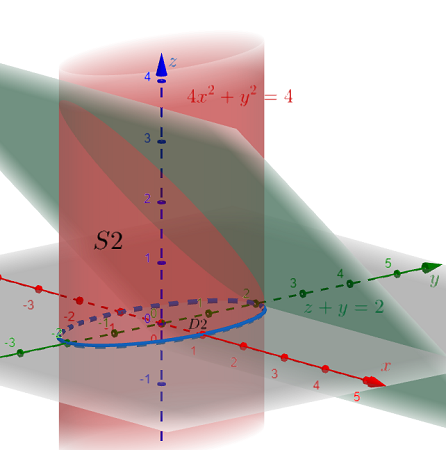# Find the volume of the solid that bounded by i) z = x^2 + y^2 and z = 1 - x^2. ii) 4 x^2 + y^2 =...

## Question:

Find the volume of the solid that bounded by

i) {eq}z = x^2 + y^2 {/eq} and {eq}z = 1 - x^2 {/eq}.

ii) {eq}4 x^2 + y^2 = 4 {/eq} and {eq}z + y = 2 {/eq}.

## Volumes of Solids:

The volume of the solid {eq}S {/eq} in {eq}\mathbb{R}^3 {/eq} can be determined by evaluating the triple integrals:

$$Volume = \iiint \limits_S dV$$

Suppose the solid {eq}S {/eq} is bounded by the smooth surfaces of the functions {eq}F(x,y) \le z \le G(x,y) {/eq} over the planar region {eq}D {/eq} which lies on the xy-plane.

Then, the triple integrals for the volume of the solid can be written as the double integrals:

$$Volume = \iint \limits_D \left[ G(x,y) - F(x,y) \right] dA$$

Furthermore, suppose the planar region {eq}D {/eq} can be defined as a Type I region, one which is bounded by continuous functions of x, such that the region is described as {eq}h_1(x) \le y h_2(x) {/eq} where {eq}a \le x \le b {/eq}.

The double integrals for the volume of the solid can then be expressed as the iterated integrals:

$$Volume = \int_a^b \int_{h_1(x)}^{h_2(x)} \left[ G(x,y) - F(x,y) \right] dy \,dx$$

The same principle can be applied to when the region {eq}D {/eq} is a Type II region, a planar region which is instead bounded by continuous functions of y over some interval/s of y.

1. Let {eq}S1 {/eq} be the solid bounded by {eq}z = x^2 + y^2 {/eq} and {eq}z = 1 - x^2 {/eq}.Additionally, let {eq}D1 {/eq} be the region on the xy-plane that is directly under the solid {eq}S1 {/eq}. Solve for the equation of the intersection of the two surfaces:

{eq}\begin{align*} &z = x^2 + y^2 = 1 - x^2 \\ &\Rightarrow x^2 + y^2 = 1 - x^2 \\ &\Rightarrow 2x^2 + y^2 = 1 & \text{[Equation of the Elliptical Region } D1 ] \end{align*} {/eq}Based on the graphs, {eq}z = 1 - x^2 \ge z = x^2 + y^2 {/eq}. This can be confirmed algebraically by taking a point in the region {eq}D {/eq} and solving for the value {eq}z {/eq} using the equations of the two surfaces.

For instance, the origin {eq}(0,0) {/eq} is in the region {eq}D {/eq}, and {eq}1 - (0)^2 = 1 > 0 = (0)^2 + (0)^2 {/eq}.

Moving on to the region of integration, express the equation of the ellipse as functions of x to define the region {eq}D1 {/eq} as a Type I region.

{eq}\begin{align*} &2x^2 + y^2 = 1 \\ &\Rightarrow y^2 = 1 - 2x^2 \\ &\Rightarrow y = \sqrt{ 1 - 2x^2 } & \text{[Equation of the Upper Semi-Ellipse]} \\ &\Rightarrow y = - \sqrt{ 1 - 2x^2 } & \text{[Equation of the Lower Semi-Ellipse]} \\ \\ &y^2 = 1 - 2x^2 = 0 & \text{[Solve for the } x \text{-intercepts]} \\ &\Rightarrow 2x^2 = 1 \\ &\Rightarrow x^2 = \frac{1}{2} \\ &\Rightarrow x = \pm \frac{1}{\sqrt{2}} \end{align*} {/eq}

Therefore, the solid {eq}S1 {/eq} can be defined as {eq}x^2 + y^2 \le z \le 1 - x^2 {/eq} over the region {eq}D1 = \left\{\, (x,y) \,|\, -\frac{1}{\sqrt{2}} \le x \le \frac{1}{\sqrt{2}} \text{ and } -\sqrt{ 1 - 2x^2 } \le y \le \sqrt{ 1 - 2x^2 } \,\right\} {/eq}.

Finally, the volume of the solid {eq}S1 {/eq} is given by the double integrals:

{eq}\begin{align*} Volume_{S1} &= \iint \limits_{D1} [ (1 - x^2) - (x^2 + y^2) ] \,dA & \text{[Evaluate as iterated integrals]} \\ &= \int_{-\frac{1}{\sqrt{2}}}^{\frac{1}{\sqrt{2}}} \int_{-\sqrt{ 1 - 2x^2 }}^{\sqrt{ 1 - 2x^2 }} ( 1 - 2x^2 - y^2 ) \,dy \,dx & \text{[Integrate with respect to } y \text{]} \\ &= \int_{-\frac{1}{\sqrt{2}}}^{\frac{1}{\sqrt{2}}} \left. \left( y - 2x^2 y - \frac{y^3}{3} \right) \right|_{-\sqrt{ 1 - 2x^2 }}^{\sqrt{ 1 - 2x^2 }} \,dx & \text{[Note that the result of the integral is an odd function. Thus, } f(-a) = - f(a) \text{ and } f(a) - f(-a) = 2f(a) ] \\ &= \int_{-\frac{1}{\sqrt{2}}}^{\frac{1}{\sqrt{2}}} 2 \left( \sqrt{ 1 - 2x^2 } - 2x^2 \sqrt{ 1 - 2x^2 } - \frac{\sqrt{ 1 - 2x^2 }^3}{3} \right) \,dx \\ &= \int_{-\frac{1}{\sqrt{2}}}^{\frac{1}{\sqrt{2}}} 2 \sqrt{ 1 - 2x^2 } \left( 1 - 2x^2 - \frac{1 - 2x^2}{3} \right) \,dx \\ &= \int_{-\frac{1}{\sqrt{2}}}^{\frac{1}{\sqrt{2}}} \frac{4}{3} (1 - 2x^2)^\frac{3}{2} \,dx & \left[ \text{Substitute } x = \frac{1}{\sqrt{2}} \cos w \text{ and } dx = \frac{-1}{\sqrt{2}} \sin w \,dw \right] \\ &= \int_{w\left(-\frac{1}{\sqrt{2}}\right)}^{w\left(\frac{1}{\sqrt{2}}\right)} \frac{4}{3} \left[ 1 - 2 \left( \frac{1}{\sqrt{2}} \cos w \right)^2 \right]^\frac{3}{2} \left( \frac{-1}{\sqrt{2}} \sin w \,dw \right) & \left[ \text{The new limits of integration are } w\left(\frac{-1}{\sqrt{2}}\right) = \cos^{-1} \sqrt{2} \left(\frac{-1}{\sqrt{2}}\right) = \cos^{-1} (-1) = \pi \text{ and } w\left(-\frac{1}{\sqrt{2}}\right) = \cos^{-1} \sqrt{2} \left(\frac{1}{\sqrt{2}}\right) = \cos^{-1} 1 = 0 \right] \\ &= \int_\pi^0 - \frac{4}{3\sqrt{2}} \sin w ( 1 - \cos^2 w )^\frac{3}{2} \,dw & \text{[Use the identity } \cos^2 \theta + \sin^2 \theta = 1 ] \\ &= \int_\pi^0 - \frac{4}{3\sqrt{2}} \sin w ( \sin^2 w )^\frac{3}{2} \,dw & \left[ \int_a^b f(x) \,dx = \int_b^a - f(x) \,dx \right] \\ &= \int_0^\pi \frac{4}{3\sqrt{2}} \sin w \sin^3 w \,dw & \left[ \text{For all constant } k \,,\, \int k \cdot f(x) \,dx = k \int f(x)\,dx \right] \\ &= \frac{4}{3\sqrt{2}} \int_0^\pi \sin^3 w \sin w \,dw & \text{[Integrate by parts. Let } u = \sin^3 w \text{ and } dv = \sin w \,dw \text{ such that } du = 3 \sin^2 w \cos w \,dw \text{ and } v = - \cos w ] \\ &= \frac{4}{3\sqrt{2}} \left[ \left. \left( - \cos w \sin^3 w \right) \right|_0^\pi - \int_0^\pi - 3 \sin^2 w \cos^2 w \,dw \right] & \text{[Fundamental Theorem of Calculus]} \\ &= \frac{4}{3\sqrt{2}} \left[ ( 0 - 0 ) + 3 \int_0^\pi \sin^2 w \cos^2 w \,dw \right] & \left[ \text{Use the identity } \sin^2 \theta \cos^2 \theta = \frac{1}{8} (1 - \cos 4\theta) \right] \\ &= \frac{4}{\sqrt{2}} \int_0^\pi \frac{1}{8} (1 - \cos 4w) \,dw & \text{[Substitute } u = 4w \text{ such that } du = 4\,dw \,,\, u(0) = 0 \text{ and } u(\pi) = 4\pi ] \\ &= \frac{4}{\sqrt{2}} \int_0^{4\pi} \frac{1}{32} (1 - \cos u) \,du \\ &= \frac{1}{8\sqrt{2}} \int_0^{4\pi} (1 - \cos u) \,du & \text{[Integrate with respect to } u \text{]} \\ &= \frac{1}{8\sqrt{2}} \left. \left( u - \sin u \right) \right|_0^{4\pi} & \text{[Fundamental Theorem of Calculus]} \\ &= \frac{1}{8\sqrt{2}} \left[ ( 4\pi - \sin 4\pi ) - ( 0 - \sin 0 ) \right] \\ &= \frac{1}{8\sqrt{2}} ( 4\pi ) \cdot \frac{\sqrt{2}}{\sqrt{2}} \\ Volume_{S1} &= \boxed{ \frac{\pi \sqrt{2}}{4} } & \boxed{ \text{Volume of the Solid } S1 } \end{align*} {/eq}

2. Let {eq}S2 {/eq} be the solid bounded by {eq}4x^2 + y^2 = 4 {/eq} and {eq}z + y = 2 {/eq}. We will assume that the problem pertains to the portion of the solid above the xy-plane {eq}(z=0) {/eq} that is bounded by the graphs of the given equations.Based on the graph, solid is bounded above by the plane {eq}z = 2 - y {/eq} and below by the region {eq}D {/eq} on the xy-plane {eq}(z=0) {/eq}, which is in turn bounded by the ellipse {eq}4x^2 + y^2 = 4 {/eq}.

Again, defining the region of integration as a Type I region, express the equation of the ellipse as functions of x:

{eq}\begin{align*} &4x^2 + y^2 = 4 \\ &\Rightarrow y^2 = 4 - 4x^2 \\ &\Rightarrow y = 2 \sqrt{ 1 - x^2 } & \text{[Equation of the Upper Semi-Ellipse]} \\ &\Rightarrow y = - 2 \sqrt{ 1 - x^2 } & \text{[Equation of the Lower Semi-Ellipse]} \\ \\ &y^2 = 4 - 4x^2 = 0 & \text{[Solve for the } x \text{-intercepts]} \\ &\Rightarrow 4x^2 = 4 \\ &\Rightarrow x^2 = 1 \\ &\Rightarrow x = \pm 1 \end{align*} {/eq}

Therefore, the solid {eq}S2 {/eq} is defined as {eq}0 \le z \le 2 - y {/eq} and over the region {eq}D2 = \left\{\, (x,y) \,|\, -1 \le x \le 1 \text{ and } -2 \sqrt{ 1 - x^2 } \le y \le 2 \sqrt{ 1 - x^2 } \,\right\} {/eq}.

Then, the volume of the solid {eq}S2 {/eq} is given by the double integrals:

{eq}\begin{align*} Volume_{S2} &= \iint \limits_{D2} [ (2 - y) - 0 ] \,dA & \text{[Evaluate as iterated integrals]} \\ &= \int_{-1}^1 \int_{-2 \sqrt{ 1 - x^2 }}^{2 \sqrt{ 1 - x^2 }} (2 - y) \,dy \,dx & \text{[Integrate with respect to } y \text{]} \\ &= \int_{-1}^1 \left. \left( 2y - \frac{y^2}{2} \right) \right|_{-2 \sqrt{ 1 - x^2 }}^{2 \sqrt{ 1 - x^2 }} & \text{[Fundamental Theorem of Calculus]} \\ &= \int_{-1}^1 \left( \left[ 2 \left( 2 \sqrt{ 1 - x^2 } \right) - \frac{1}{2} \left( 2 \sqrt{ 1 - x^2 } \right)^2 \right] - \left[ 2 \left( -2 \sqrt{ 1 - x^2 } \right) - \frac{1}{2} \left( -2 \sqrt{ 1 - x^2 } \right)^2 \right] \right) \,dx \\ &= \int_{-1}^1 \left[ \left( 4 \sqrt{1 - x^2} - 2 + 2x^2 \right) - \left( - 4 \sqrt{1 - x^2} - 2 + 2x^2 \right) \right] \,dx \\ &= \int_{-1}^1 8 \sqrt{1 - x^2} \,dx & \text{[Substitute } x = \cos u \text{ such that } dx = - \sin u \,du \,,\, u(-1) = \cos^{-1} (-1) = \pi \text{ and } u(1) = \cos^{-1} 1 = 0 ] \\ &= \int_\pi^0 -8 \sin u \sqrt{1 - \cos^2 u} \,du & \text{[Use the identity } \cos^2 \theta + \sin^2 \theta = 1 ] \\ &= \int_\pi^0 -8 \sin u \sqrt{ \sin^2 u } \,du & \left[ \int_a^b f(x) \,dx = \int_b^a - f(x) \,dx \right] \\ &= \int_0^\pi 8 \sin^2 u \,du & \left[ \text{Use the identity } \sin^2 \theta = \frac{1}{2} (1 - \cos 2\theta) \right] \\ &= \int_0^\pi 4 (1 - \cos 2u) \,du & \text{[Substitute } w = 2u \text{ such that } dw = 2\,du \,,\, w(0) = 0 \text{ and } w(\pi) = 2\pi ] \\ &= \int_0^{2\pi} 2 (1 - \cos w) \,dw \\ &= 2 \int_0^{2\pi} (1 - \cos w) \,dw & \text{[Integrate with respect to } w \text{]} \\ &= 2 \left. \left( w - \sin w \right) \right|_0^{2\pi} & \text{[Fundamental Theorem of Calculus]} \\ &= 2 [ (2\pi - \sin 2\pi) - (0 - \sin 0) ] \\ &= 2 (2\pi) \\ Volume_{S2} &= \boxed{ 4\pi } & \boxed{ \text{Volume of the Solid } S2 } \end{align*} {/eq}